# Electronics and Communication Engineering - Microwave Communication

### Exercise :: Microwave Communication - Section 2

26.

For a circular wave guide

 A. cutoff frequency for TE10 and TE01 modes are same B. cutoff frequency for TE10 and TE01 modes are different C. cutoff frequency for TE10 mode = twice the cutoff frequency for TE20 mode D. cutoff frequency for TE10 mode = haf the cutoff frequency for TE20 mode

Answer: Option A

Explanation:

When a circular waveguide is rotated by 90°, the configuration remain the same.

27.

In the given figure the reflected voltage wave after first reflection is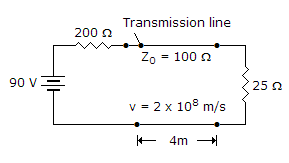A. 18 V B. -18 V C. 30 V D. -30 V

Answer: Option B

Explanation: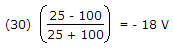.

28.

Assertion (A): A half wavelength line can be used as a 1 : 1 transformer.

Reason (R): The input impedance of a half wavelength line is equal to load impedance.

 A. Both A and R are correct and R is correct explanation of A B. Both A and R are correct but R is not correct explanation of A C. A is correct but R is wrong D. A is wrong but R is correct

Answer: Option A

Explanation:

Since a half wavelength line has an input impedance equal to load impedance, the impedance transformation ratio is 1:1.

29.

The magnitudes of OC and SC input impedances of a transmission line an 100 Ω and 25 Ω. The characteristic impedance is

 A. 25 Ω B. 50 Ω C. 75 Ω D. 100 Ω

Answer: Option B

Explanation: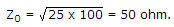30.

A loss less line is terminated in a circular lines are E lines

 A. SWR = 0 B. SWE = 0 C. SWR is finite D. SWR = ∞

Answer: Option C

Explanation: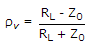= finite number and VSWR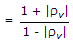= finite number.

#### Current Affairs 2021

Interview Questions and Answers